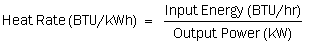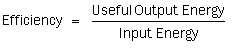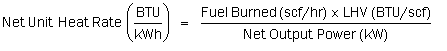What is heat rate? Why is it so important? These are very important questions with critical answers that everyone that manages, operates, or maintains a combined cycle power plant should know.

In this article, we are going to find out what heat rate is. We will discuss the various components at the plant and how they affect heat rate. Most importantly, we will find out how each and every one of you can help improve your plants heat rate and how this can save the plant a considerable amount of money. This article describes heat rate and heat rate deviation. It also calculates the impact of heat rate deviation.

## What is Heat Rate?

Heat rate is the common measure of system efficiency in a steam power plant. It is defined as “the energy input to a system, typically in Btu/kWh, divided by the electricity generated, in kW.” Mathematically:Efficiency is “a ratio of the useful energy output by the system to the energy input to the system.” Mathematically:As you can see, heat rate is simply the inverse of efficiency. With that in mind, if you increase plant efficiency, which is good, then you would lower heat rate. What this means is that the lower the heat rate, the better your plant is running and, therefore, the more competitive it is.

As you can see by the equations, efficiency has no units, but heat rate does. That is because with heat rate, you typically are measuring the chemical energy input and the electrical energy output, and this will leave you with the units of BTU/kWh.

### Plant Heat Rate

Plant heat rate is a measure of the combined performance of the gas turbine cycle, the steam turbine cycle, and any other associated auxiliaries. This may include more than one gas turbine and steam turbine. Heat rate can be further divided to compare units at the same plant, where you might compare Power Block 1 to Power Block 2. You may even use heat rate to compare to other generating units at different sites. Caution must be taken when comparing different types of generating stations, as their chemical energy input may be quite different from yours.

When calculating plant heat rate, the energy input to the system is the chemical energy in the fuel.

Chemical Energy of Fuel = Total Fuel Used (scf/hr) x Higher Heating Value (HHV)(BTU/scf)

The power generated is simply the gross or net generation in kW.

• If gross generation is used, then the resultant heat rate is the gross unit heat rate.
• If net generation is used, then the resultant heat rate is net unit heat rate.

By substituting from the previous equations we get:Unit analysis is very important. At many sites, fuel flow is measured in lbm/hr, and HHV is given in scf/hr. It is very important to make sure that you match units when doing these problems. At some sites, there is a chromatograph that will measure the heat content of the fuel, and your DCS will convert the fuel flow and HHV to MMBTU. It is very important you make sure you are using the correct units in your equations.

### Conversion Factors for Standard Cubic Foot to Pounds Mass

.0458 lbm per scf or 21.826 scf per lbm

Practice Problem:

Calculate plant heat rate for the following conditions (GT CC):As you will notice, there is a 4.1% difference between gross heat rate and net heat rate. This shows you the importance of maintaining site loads at a minimum. That will increase your net plant output and, thus, lower your heat rate.

An important point to make here is that the percentage the plant is loaded has a factor on heat rate. The closer the plant is to base load, the better the heat rate. This is due to many factors. The gas turbine is tuned to operate more efficiently at base load. Additionally, the steam turbine will be more efficient at base load. Also, you normally still have the same house loads at minimum load as you do at base load, or they are not proportionally lowered.

It is very important that your site marketing team understands this and understands the consequences financially of dispatching the units in less than full load configuration.

### Why is Heat Rate Important?

Heat rate and thermal performance improvement are integral parts of any serious effort for cost containment in an electric generating station. As the electric power industry expands deregulation and competition, cost containment and the ability to provide energy at the lowest possible cost become important issues. The power producer must provide a lower-cost energy product than the competition and yet still remain profitable in the long term. Fuel-cost reduction and increased reliability and availability through efficiency improvement are key methods of improving profitability.

In many generating stations, fuel expenditure is as high as 90% of the total operations budget. As a result, a 1% reduction in fuel usage achieved through heat rate improvements has a significant, positive impact on profitability. For a 500 MW combined cycle unit, the annual fuel savings could easily exceed \$1,500,000 by simply improving heat rate by 200 units.

Heat rate improvement requires the support of personnel at all levels, in addition to support from both station and corporate management. By monitoring and acting on many of the items identified here and decreasing the amount of controllable losses, plant heat rate can be improved to an optimum level and maintained at that optimum level.

Controllable losses, often called operator controllable losses, are defined as “those heat rate losses that can be directly impacted (either positively or negatively) by the actions of the operator.” In many cases, the actual “control” is handled by the control system, but often, operator intervention can impact the magnitude of the loss.

### Heat Rate Deviation

Most power plants have a target or design heat rate that they are trying to achieve. If the actual heat rate does not match the target, the difference between the actual and target heat rate is the heat rate deviation. Most heat rate deviations are often small in relation to the overall heat rate. For instance, a 70 Btu/kWh deviation is only 1% of a 7,000 Btu/kWh heat rate that is normal for a combined cycle plant.

This does not mean much to most people; however, if we calculate the fuel cost associated with the heat rate deviation, this puts in perspective what this is really costing the plant. Once employees see the dollar figure attached to the losses, it is easier to get all personnel involved in trying to improve heat rate. This is especially important in the corporate budgeting process.

If two projects have been proposed for two different units and each project will improve unit heat rate by 75 Btu/kWh, which project has the best payback? If the units have similar heat rates, similar fuel costs, and are similar in size, the payback will be nearly equal. However, if one unit is 525 MW and the other is 215 MW, then which project has the shortest payback? Without performing the cost calculation, it may be impossible to know. That is one of the primary uses of the heat rate cost calculations. When using heat rate information for making business decisions, it is important to realize that heat rate is only one component in the decision-making process.

Other considerations include system reliability, emissions, maintenance costs, operations costs, and safety concerns. For example, it would not be a good economic decision to install a piece of equipment to improve heat rate if the maintenance cost of the equipment was more than the heat rate savings.

### Cost of Heat Rate Deviations

The cost of a heat rate increase can be directly tied to the cost of fuel. Heat rate is expressed in units of Btu/kWh, and fuel is usually purchased in quantities of million Btus. A reduction in heat rate is simply a reduction in the quantity of fuel necessary to produce a fixed number of kWh.

To calculate heat rate deviation cost, it is important to understand that several factors need to be known and understood. Those include gross capacity, auxiliary loads, fuel cost, and the unit’s capacity factor. What we are going to calculate is the cost of 1 Btu/kWhr, and then from there, we can determine what savings can be had by an improvement or what something is costing the facility in lost heat rate. We are going to relate this to one year of operation so it can be seen what this cost is per year. This will give you the dramatic look at what savings and losses can be had with minor deviations.

Annual Fuel Cost = Heat Rate Deviation (HRD) x Fuel Cost (FC) x Capacity Factor (CF) x [Gross Capacity (GC) Auxiliary Loads (AL)] x Time (Number of Hours in a Year)

Since we will be calculating for what one unit of heat rate costs, we will substitute 1 BTU/kWhr for our heat rate deviation.

Gas Turbine Combined Cycle Calculation

Other assumptions:This is the annual cost for one unit of heat rate deviation. With that said, if you do just a 0.5% increase in deviation on a plant that has a heat rate of 7,400, this will be a 37-unit heat rate improvement, which calculates to an annual fuel savings of \$349,267.05. This number usually gets the attention of most of the people at the plant.

You will be able to use this equation when calculating savings for your plant on various projects.

As an example, a project was conducted on a combined cycle facility where several drain valves on the HRSG were leaking during operation. It was determined that this was causing a loss in heat rate due to the loss in steam. The project was to replace the drain valves with better-quality metal-seated ball valves. This project cost was \$15,000 per unit. Since the owners were unsure that there would be a cost benefit seen, they would only allow one unit to be done. Once the results on the heat rate improvement were documented, they would decide if it was cost-effective to do the other unit. Once the valves were replaced on the unit, the heat rate on the unit was improved by 11 Btu/kWhr. The cost for one Btu/kWhr was \$8,321. This showed an annual fuel savings of \$91,531. The payback on this project was less than two months. With this ammunition in the bag, a presentation was made to the owner, and the other unit had the project completed during the next outage.

Making a presentation to any level of management goes a long way when the cost savings can be seen.